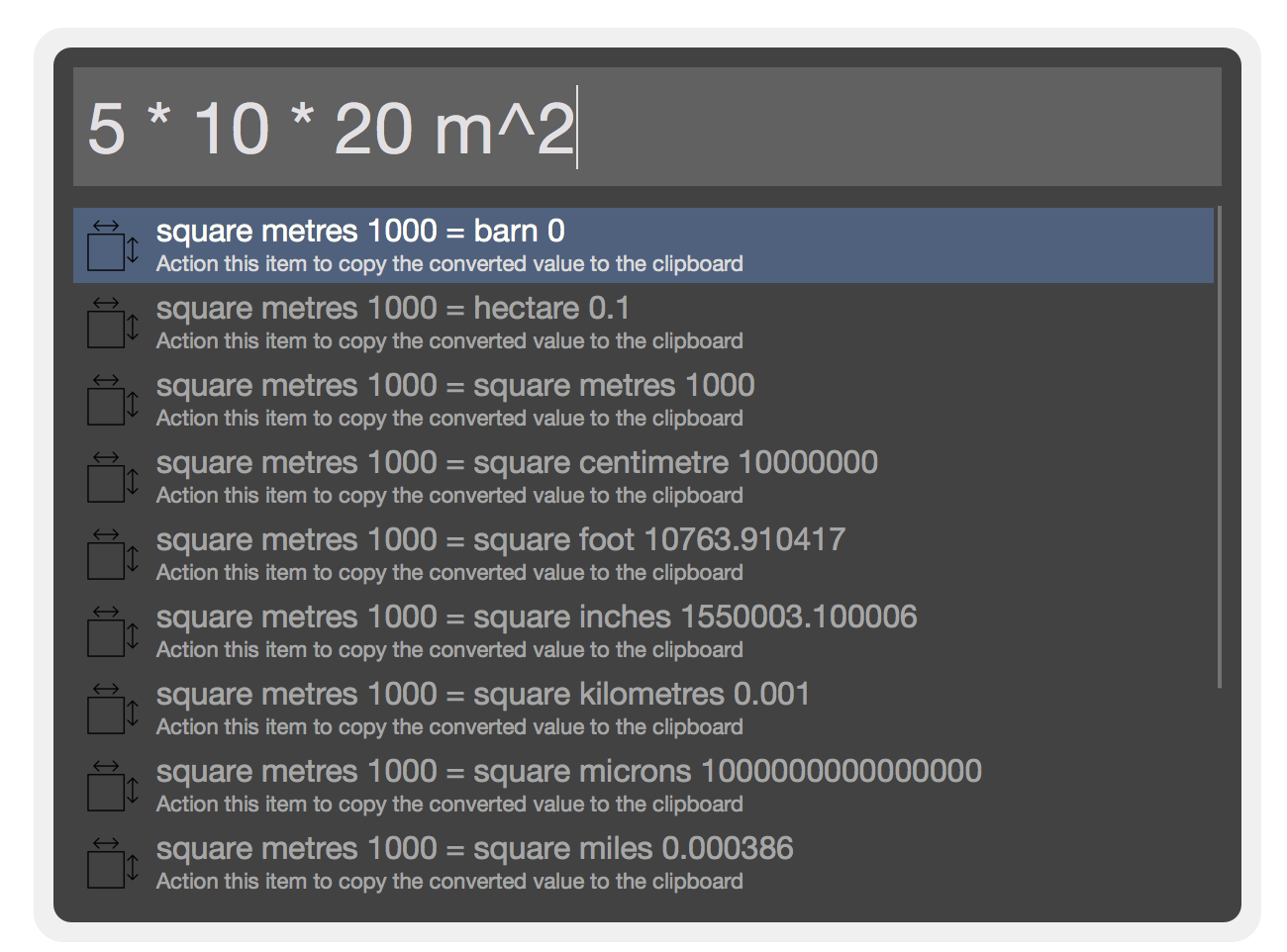Unit converter

Short Description

Simple tool to convert measurements with advanced calculation support

02 Jun 2019

Compatibility

 Yosemite(10.10) Mavericks(10.9) Mountain Lion(10.8) Lion(10.7) Snow Leopard(10.6)Categories

Alfred unit converter is a smart calculator for Alfred with support for unit conversions to make it a bit comparable to the Google Calculator and Wolfram Alpha.

If new units and/or other names for units should be added please let me know by creating an issue at: https://github.com/WoLpH/alfred-converter/issues

Example queries

1m in cm # Just a simple conversion
2^30 byte # Using powers before conversion
5' # Converting units with special characters
5'6" # Converting combined units with special characters
20" # Like above
5 * cos(pi + 2) # Executing mathematical functions
5 * pi + 2 mm in m # Mathematical constants with unit conversion
1 * cos(pi/2) - sin(pi^2) # More advanced mathematical expressions
ln(e^10) # Testing the ln(x) alias of log _e(x)
log(e^10) # The normal log method
5+3^2" in mm # Testing math with unit conversion
1 + 2 / 3 * 4) mm^2 in cm^2 # Unbalanced paranthesis with unit conversion
((1 + 2 / 3 * 4) mm^2 in cm^2 # Unbalanced paranthesis the other way
inf - inf # Not actually possible, but we backtrack to "inf"
0b1010 + 0xA - 050